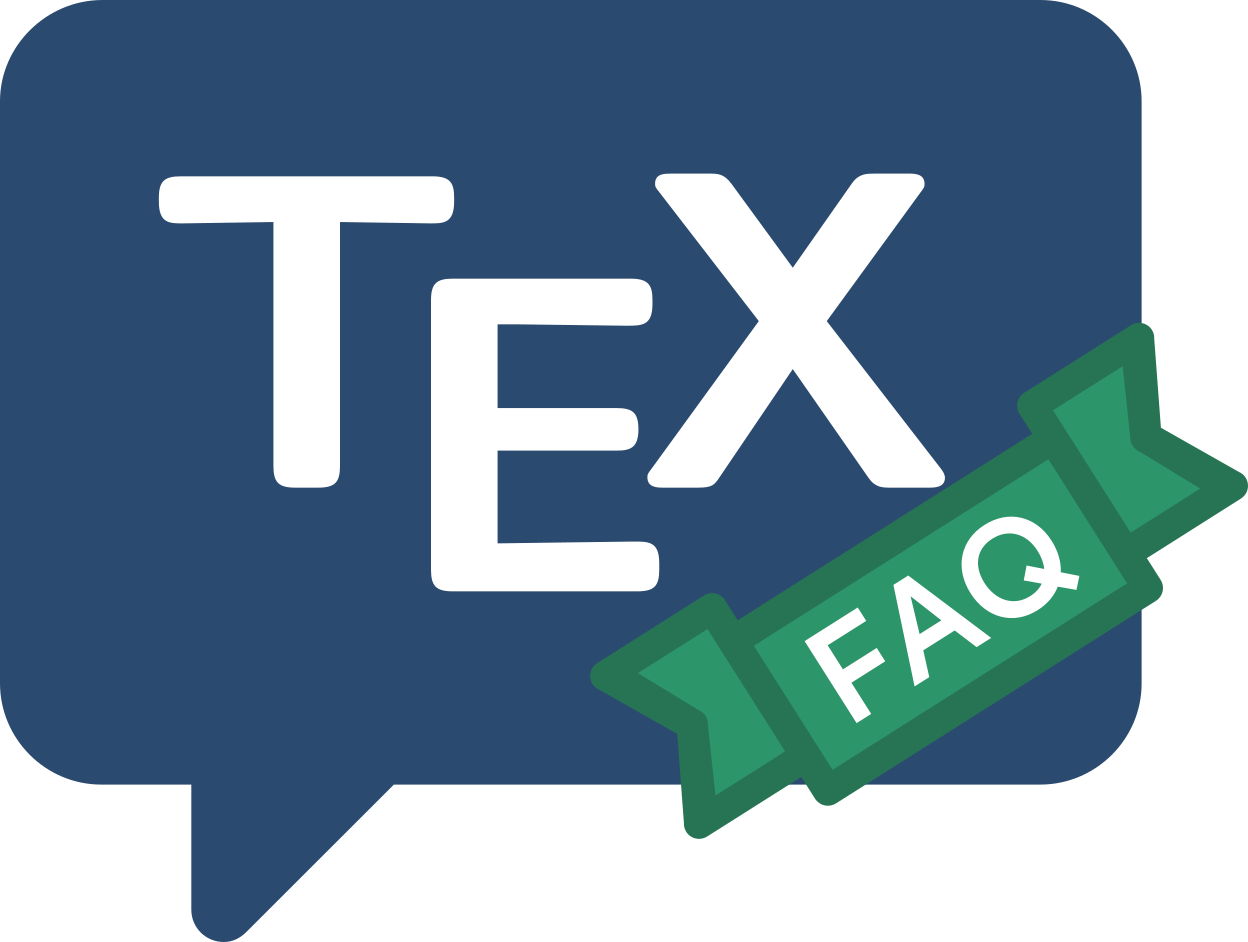Frequently Asked Question List for TeX

# LaTeX gets cross-references wrong

Sometimes, however many times you run LaTeX, the cross-references are just wrong. A likely reason is that you have placed the label before the data for the label was set; if the label is recording a \caption command, the \label command must appear after the \caption command, or be part of it. For example:

\begin{figure}
<the illustration itself>
\caption{My figure}
\label{myfig}
\end{figure}


is correct, as is

\begin{figure}
<the illustration itself>
\caption{My figure%
\label{myfig}}
\end{figure}


whereas, in

\begin{figure}
<the illustration itself>
\label{myfig}
\caption{My figure}
\end{figure}


the label will report the number of the section (or whatever) in which the surrounding text resides, or the like.

You can, with the same malign effect, shield the \caption command from its associated \label command, by enclosing the caption in an environment of its own. This effect will be seen with:

\begin{figure}
<the illustration itself>
\caption{A Figure}
\end{figure}
\label{myfig}


where the \label definitely is after the \caption, but because the figure environment closed before the \label command, the \caption is no longer “visible”.

In summary, the \label must be after the command that defines it (e.g., \caption), and if the \caption is inside an environment, the \label must be in there too.

FAQ ID: Q-crossref